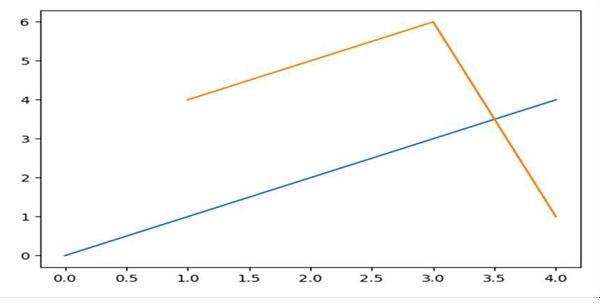# What is the currently correct way to dynamically update plots in Jupyter/iPython?

We can first activate the figure using plt.ion() method. Then, we can update the plot with different sets of values.

## Steps

• Create fig and ax variables using subplots method, where default nrows and ncols are 1.

• Draw a line, using plot() method.

• Set the color of line, i.e., orange.

• Activate the interaction, using plt.ion() method.

• To make the plots interactive, change the line coordinates.

## Example

In : %matplotlib auto

Using matplotlib backend: GTK3Agg

In : import matplotlib.pyplot as plt

# Diagram will get popped up. Let’s update the diagram.
In : fig, ax = plt.subplots()

# Drawing a line
In : ax.plot(range(5))

In : plt.ion()

# updating figures with new lines.
In : ax.plot([1, 3, 4], [4, 6, 1])

## Output